# 33 Year NEET Previous Year Questions: Moving Charges & Magnetism - 1

## 25 Questions MCQ Test Topic-wise MCQ Tests for NEET | 33 Year NEET Previous Year Questions: Moving Charges & Magnetism - 1

Description
Attempt 33 Year NEET Previous Year Questions: Moving Charges & Magnetism - 1 | 25 questions in 50 minutes | Mock test for NEET preparation | Free important questions MCQ to study Topic-wise MCQ Tests for NEET for NEET Exam | Download free PDF with solutions
QUESTION: 1

### A current carrying coil is subjected to a uniformmagnetic field. The coil will orient so that itsplane becomes 

Solution:

The plane of coil will orient itself so that area vector aligns itself along the magnetic field.
So, the plane will orient perpendicular to the magnetic field.

QUESTION: 2

### Tesla is the unit of               

Solution:

Tesla is the unit of magnetic field.

QUESTION: 3

### Energy in a current carrying coil is stored in theform of             

Solution:

Energy is stored in magnetic field.

QUESTION: 4

The total charge induced in a conducting loopwhen it is moved in a magnetic field depends on         

Solution: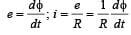Total charge induced =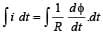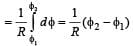QUESTION: 5

The magnetic induction at a point P which is at adistance of 4 cm from a long current carryingwire is 10–3 T. The field of induction at a distance12 cm from the current will be 

Solution: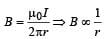As the distance is increased to three times, the magnetic induction reduces to one third.
Hence,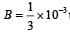tesla = 3.33 x 10-4 tesla

QUESTION: 6

A deuteron of kinetic energy 50 keV is describinga circular orbit of radius 0.5 metre in a planeperpendicular to the magnetic field B. The kineticenergy of the proton that describes a circularorbit of radius 0.5 metre in the same plane withthe same B is        

Solution:

For a charged particle orbiting in a circular path in a magnetic field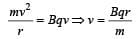or, mv2 = Bqvr

Also,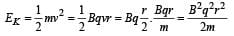For deuteron,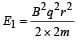For proton,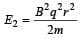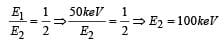QUESTION: 7

A uniform magnetic field acts at right angles tothe direction of motion of electron. As a result,the electron moves in a circular path of radius2cm. If the speed of electron is doubled, thenthe radius of the circular path will be 

Solution: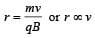As v is doubled, the radius also becomes double. Hence, radius = 2 × 2 = 4 cm

QUESTION: 8

The magnetic field at a distance r from a longwire carrying current i is 0.4 tesla. The magneticfield at a distance 2r is                

Solution: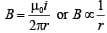When r is doubled, the magnetic field becomes half, i.e., now the magnetic field will be 0.2 T.

QUESTION: 9

A straight wire of length 0.5 m that is carrying a current of 1.2 A is placed in a uniform magnetic field of induction 2T, and the magnetic field is perpendicular to the length of the wire. What is the force of the wire?

Solution:

F = Biℓ = 2 ×1.2 × 0.5 = 1.2 N

QUESTION: 10

To convert a galvanometer into an ammeter, oneneeds to connect a        

Solution:

To convert a galvanometer into an ammeter, one needs to connect a low resistance in parallel so that maximum current passes through the shunt wire and ammeter remains protected

QUESTION: 11

A coil carrying electric current is placed inuniform magnetic field, then         

Solution:

A current carrying coil has magnetic dipole moment. Hence, a torque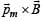acts on it in magnetic field.

QUESTION: 12

A charge moving with velocity v in X-directionis subjected to a field of magnetic induction innegative X-direction. As a result, the charge will              

Solution:

The force acting on a charged particle in magnetic field is given by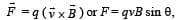When angle between v and B is 180°, F = 0

QUESTION: 13

An electron enters a region where magnetic field(B) and electric field (E) are mutually perpendicular,then        

Solution:

When the deflection produced by electric field is equal to the deflection produced by magnetic field, then the electron can go undeflected.

QUESTION: 14

A straight wire of diameter 0.5 mm carrying acurrent of 1 A is replaced by another wire of 1mm diameter carrying same current. The strengthof magnetic field far away is          [1995, 97, 99]

Solution: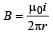and so it is independent of thickness.
The current is same in both the wires, hence magnetic field induced will be same.

QUESTION: 15

At what distance from a long straight wirecarrying a current of 12 A will the magnetic fieldbe equal to 3×10-6Wb /m2 ?        

Solution:

Current (I) = 12 A and magnetic field (B) = 3 × 10–5 Wb/m2. Consider magnetic field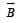at distance r..

Magnetic field ,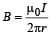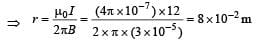QUESTION: 16

The magnetic field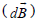due to a small element (dℓ ) at a distance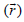and element carrying current i is           

Solution:

According to Biot Savart law,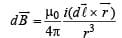QUESTION: 17

A 10 eV electron is circulating in a plane at rightangles to a uniform field at magnetic induction10– 4 Wb/m2 (= 1.0 gauss). The orbital radius ofthe electron is 

Solution:

K.E. of electron = 10 eV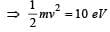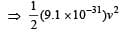= 10 x 1.6 x 10 -19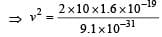⇒ v2 = 3.52 × 1012 ⇒ v = 1.88 × 106 m

Also, we know that for circular motion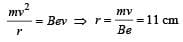QUESTION: 18

Two equal electric currents are flowing perpendicular to each other as shown in the figure. AB and CD are perpendicular to each other and symmetrically placed with respect to the current flow. Where do we expect the resultant magnetic field to be zero?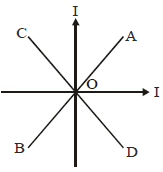Solution: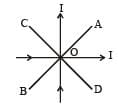Net magnetic field on AB is zero because magnetic field due to both current carrying wires is equal in magnitude but opposite in direction.

QUESTION: 19

A beam of electrons is moving with constantvelocity in a region having simultaneousperpendicular electric and magnetic fields ofstrength 20 Vm–1 and 0.5 T respectively at rightangles to the direction of motion of the electrons.Then the velocity of electrons must be         

Solution:

The electron moves with constant velocity without deflection. Hence, force due to magnetic field is equal and opposite to force due to electric field.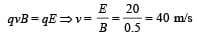QUESTION: 20

A galvanometer of resistance 20 Ω gives fullscale deflection with a current of 0.004 A. Toconvert it into an ammeter of range 1 A, therequired shunt resistance should be          

Solution:

Maximum current which can pass through galvanometer,  Ig = 0.004A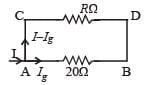Let R be the resistance of shunt.
We know potential drop across AB = Potential drop across CD R (I – Ig) = Ig (20)
⇒ R (1 – 0.004) = 0.004 × 20 ⇒ R = 0.08Ω

QUESTION: 21

A positively charged particle moving due eastenters a region of uniform magnetic field directedvertically upwards. The particle will      

Solution:

In a perpendicular magnetic field, the path of a charged particle is a circle, and the magnetic field does not cause any change in energy.

QUESTION: 22

Two long parallel wires are at a distance of 1metre. Both of them carry one ampere of current.The force of attraction per unit length betweenthe two wires is 

Solution: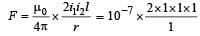= 2 × 10–7 N/m. [This relates to the definition of ampere]

QUESTION: 23

A galvanometer having a resistance of 8 ohmsis shunted by a wire of resistance 2 ohms. If thetotal current is 1 amp, the part of it passingthrough the shunt will be          

Solution: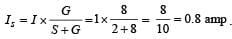QUESTION: 24

A coil of one turn is made of a wire of certainlength and then from the same length a coil oftwo turns is made. If the same current is passedin both the cases, then the ratio of the magneticinductions at their centres will be 

Solution:

Let ℓ be length of wire.
Ist case : ℓ =2πr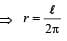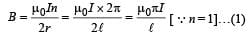2nd Case : ℓ = 2(2πr')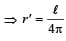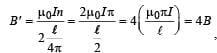using (1) (where n = 2)

QUESTION: 25

Magnetic field intensity at the centre of a coil of50 turns, radius 0.5 m and carrying a current of 2A is       

Solution:

We know that magnetic field at the centre of circular coil,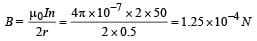Use Code STAYHOME200 and get INR 200 additional OFF Use Coupon Code